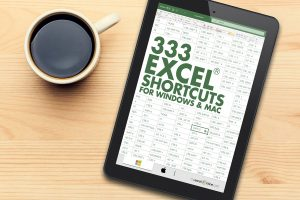There is no explicit Between formula in Excel, however, we can come up with creative ways to create this functionality. Our goal is to evaluate if a given value is between a range, for example, is 6 between 1 and 10?

We have three possible scenarios:

#### You can go through this YouTube video to understand how to use Between formula in Excel.### Between formula in Excel for Numbers

OPTION 1:Using a combination of MIN, MAX & AND function

In the example below, you have the start of the range in Column A, end of the range in Column B and the value to be evaluated in Column C.

You need to check whether the number entered in Column C is in between the numbers in Column A & Column B using a creatively formulated BETWEEN formula in Excel.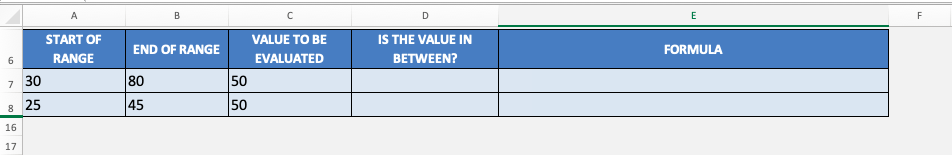The function that can be used to determine if the value in cell D7 is in-between values in cell A7 & B7 is

## =IF(AND(C7>=MIN(A7,B7),C7<=MAX(A7,B7)), “Yes”, “No”)

Let’s break this formula into parts to understand it better:

• C7 >= MIN(A7, B7) –  This expression checks whether the value in cell C7 is greater than (or equal to) the smaller of the two numbers in cell A7 and B7.
• C7 <= MAX(A7, B7) –  This expression checks whether the value in cell C7 is smaller than (or equal to) the larger of the two numbers in cell A7 and B7.
• AND( C7 >= MIN(A7, B7), C7 <= MAX(A7, B7)) – AND function simply checks whether the above two conditions are met or not i.e. whether the value in cell C7 is greater than (or equal to) the smaller number and less than (or equal to) the larger number.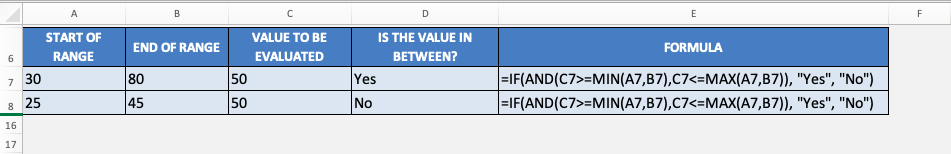OPTION 2:Using a MEDIAN function

You can use a simpler version of this complicated function by creatively using the Median formula: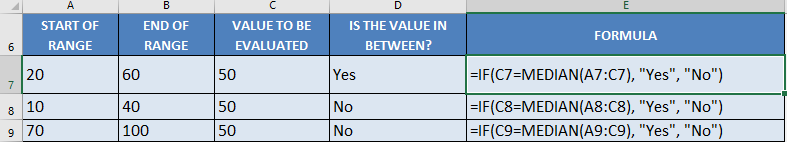## =IF(C7=MEDIAN(A7:C7), “Yes”, “No”)

In our first example above, the range is 20-60, upon checking the value 50, it is in between this range.

The median formula will return the value in the middle of these 3 values when arranged in increasing order: 20, 50, 60. The median value is 50.

Since it matches the value we are evaluating, then the answer we get is a Yes, this value (50) is in between the range.

Now that you have learned how to use Excel if between two numbers, let’s move forward to dates and text.

### Between formula in Excel for Dates

Irrespective of how you format a cell to display a date, Excel always stores it as a number. The number stored for each date actually represents the number of days since 0-Jan-1990.

1st Jan 1990 is stored as 1 (representing 1 day since 0-Jan-1990) and 23rd June 2020 is stored as 44,005 (representing 44005 days since 0-Jan-1990).

So, to check whether a date is in between two mentioned dateswe have the same application as the median formula.

Below is an example of how to use the median function to check dates.

## =IF(C10=MEDIAN(A10:C10), “Yes”, “No”)

In our first example above, the range is May 1 – July 1, upon checking the date June 1, it is in between this range.

The median formula will return the value in the middle of these 3 dates when arranged in increasing order: May 1, June 1, July 1. The median value is June 1.

Since it matches the value we are evaluating, then the answer we get is a Yes, this value (June 1) is in between the range.

### Between formula in Excel for Text

For text, we are checking if the value is alphabetically in the middle. We will be using the and formula: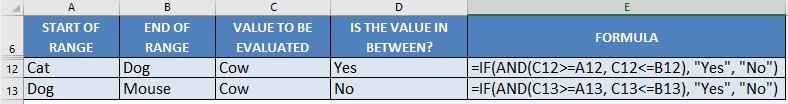## =IF(AND(C12>=A12, C12<=B12, “Yes”, “No”)

Interestingly enough, you can compare texts using the >= and <= operators.  Excel is able to compare them which goes alphabetically first or last.

In our first example above, the range is Cat – Dog, upon checking the text Cow, it is in between this range. As when arranged alphabetically, it would be: Cat, Cow, Dog.

The And formula checks if Cow >= Cat, and Cow <= Dog. You will see that both of these are true, as Cow is alphabetically later than Cat, while Cow is alphabetically ahead of Dog. Which is why we get a Yes result.

### Conclusion

In this tutorial, you have learned how to use Between formula in Excel even when there is no explicit formula available to do this. You can use a combination of various other available function to create Excel if between range functionality.

You can use MIN, MAX, MEDIAN & AND functions to create a creative Between function in Excel for numbers, dates and text.